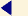back to menu STRUCTURAL DESIGN - PART 13 English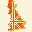18. Diffusion of the thrust at the abutments The F.E. model includes, as described above, the abutments up to a distance of 6 m from the springers of the arch, so that it allows to investigate how the thrusts diffuse into the abutments. The stresses induced by the thrusts, under the dead and live loads, are shown for each abutment at many sections. For each abutment the stresses sx (where the axis x is longitudinal) on four vertical sections and the stresses sy (where the axis y is vertical) on ten horizontal sections are shown. The distance of each section from the springer is also given.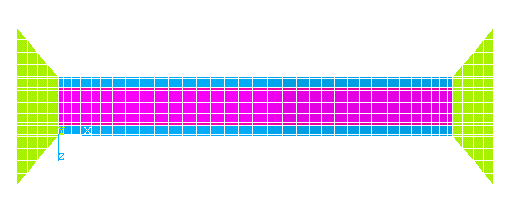fig. 60 - FE model used to calculate the diffusion of the thrust into the abutments The diffusion of the thrust at the abutments was determined for two load combinations: combination no. 1 B combination no. 5 C It is interesting to notice that in the combination 1B, when only the permanent loads and the uniform live loads act on the bridge, the maximum vertical stresses are localised in the region immediately close to the springer. In the combination 5C the maximum stresses are shifted towards the restraints at the end of both the abutments. In all the sections the maximum tensile stress is lower than the prefixed limit of 0.5 kg / cm2, in fact in all the analyses the regions of the model subject to higher tractions were disconnected (simulating the formation of cracks).   19. Stresses sx - Left abutment - Load combination no. 1B Left abutment Vertical Section at 82 cm (load combination no. 1B) - (sx in kg / cm2)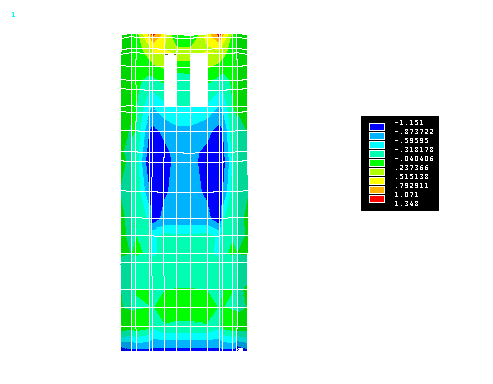Left abutment Vertical Section at 182 cm (load combination no. 1B) - (sx in kg / cm2)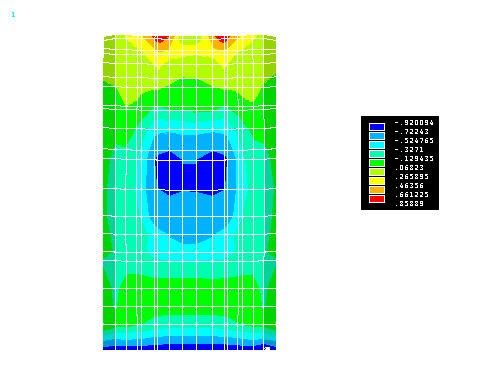Left abutment Vertical Section at 241 cm (load combination no. 1B) - (sx in kg / cm2)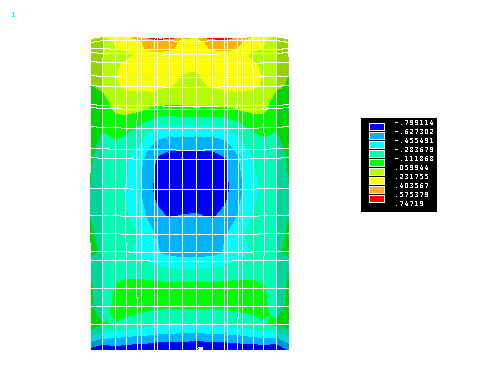Left abutment Vertical Section at 300 cm (load combination no. 1B) - (sx in kg / cm2)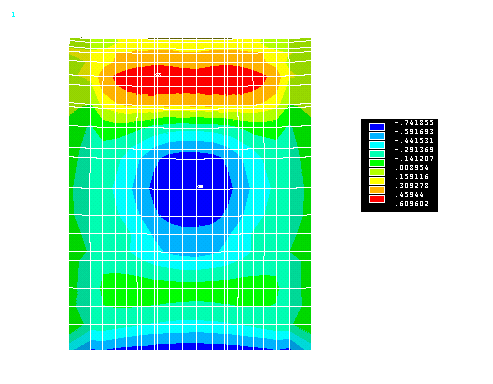Left abutment Vertical Section at 400 cm (load combination no. 1B) - (sx in kg / cm2)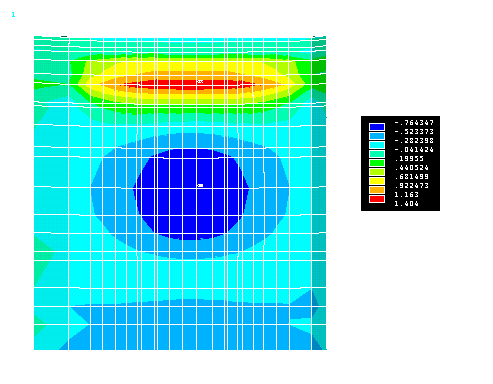Left abutment Vertical Section at 500 cm (load combination no. 1B) - (sx in kg / cm2)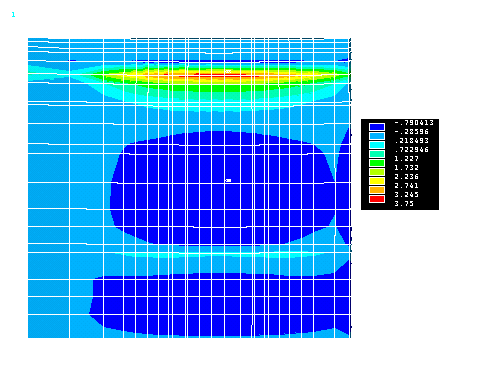Left abutment Vertical Section at 600 cm (load combination no. 1B) - (sx in kg / cm2)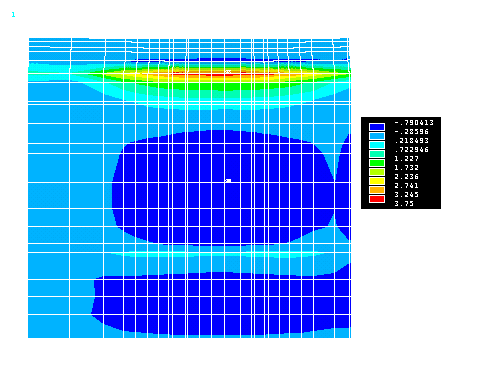Horizontal reactions at the left abutment (Load combination 1B)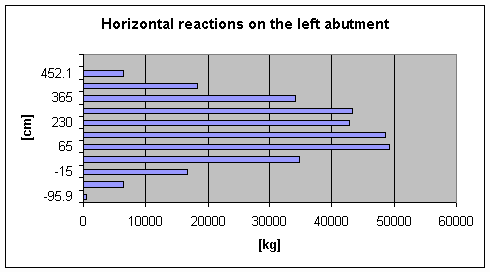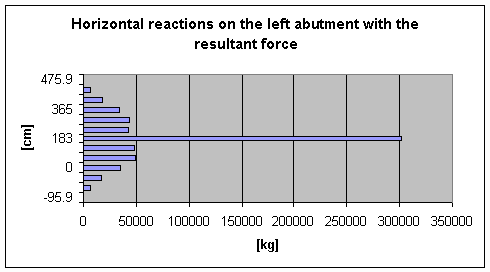In the above diagrams the level z = 0 corresponds to the level of the arch left springer. The values of the horizontal reactions were evaluated at the initial section of the abutment.   Vertical reactions at the left abutment (Load combination 1B)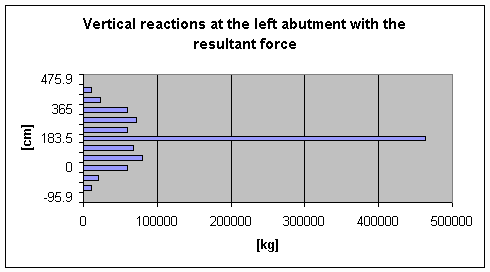In the above diagrams the level z = 0 corresponds to the level of the arch left springer. The values of the vertical reactions were evaluated at the initial section of the abutment.   20. Stresses sx - Right abutment - Load combination no. 1B Right abutment Vertical Section at 82 cm (load combination no. 1B) - (sx in kg / cm2)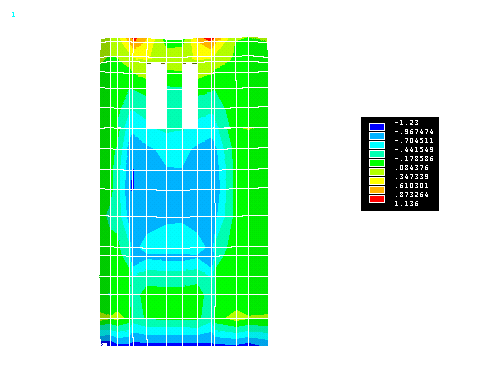Right abutment Vertical Section at 179 cm (load combination no. 1B) - (sx in kg / cm2)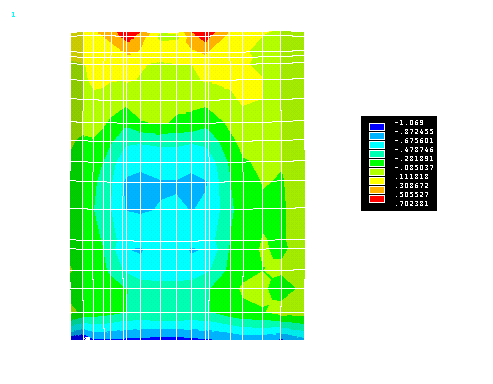Right abutment Vertical Section at 240 cm (load combination no. 1B) - (sx in kg / cm2)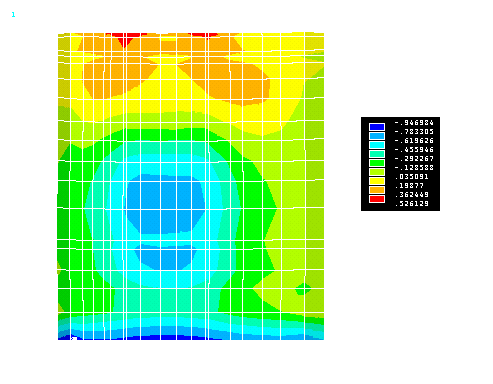Right abutment Vertical Section at 300 cm (load combination no. 1B) - (sx in kg / cm2)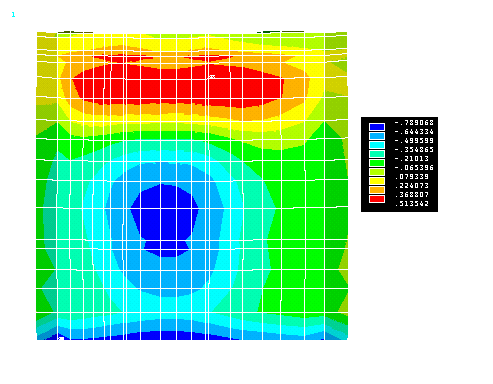Right abutment Vertical Section at 400 cm (load combination no. 1B) - (sx in kg / cm2)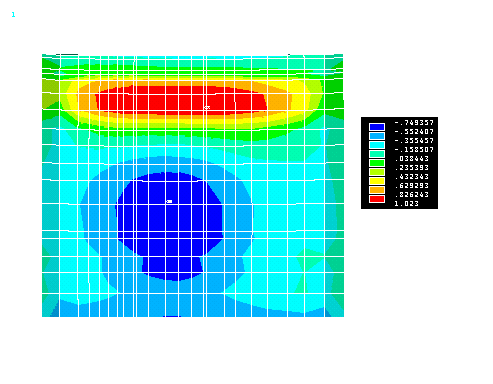Right abutment Vertical Section at 500 cm (load combination no. 1B) - (sx in kg / cm2)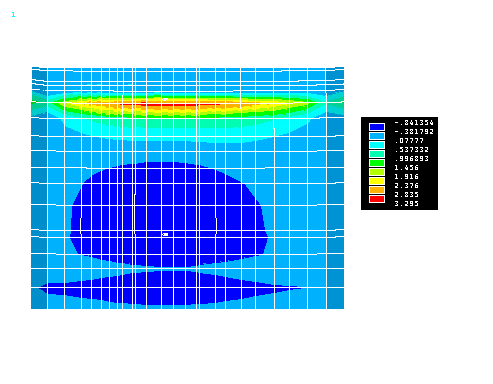Right abutment Vertical Section at 600 cm (load combination no. 1B) - (sx in kg / cm2)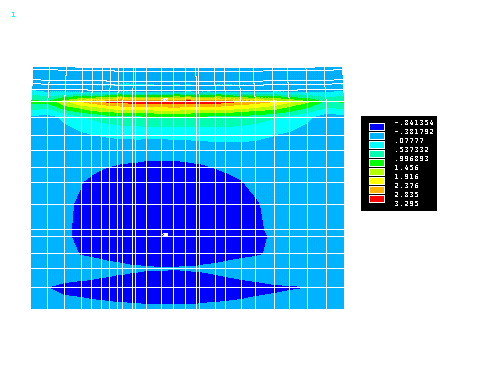Horizontal thrust at the right abutment (Load combination 1B)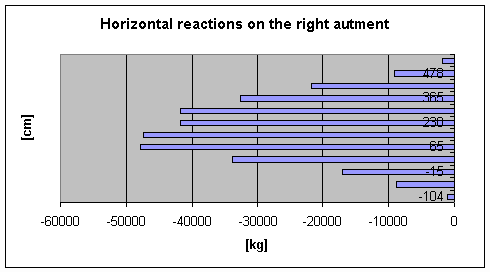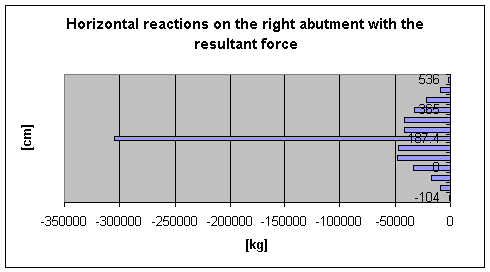In the above diagrams the level z = 0 corresponds to the level of the arch right springer. The values of the horizontal reactions were evaluated at the initial section of the abutment.   Vertical reactions at the right abutment (Load combination 1B)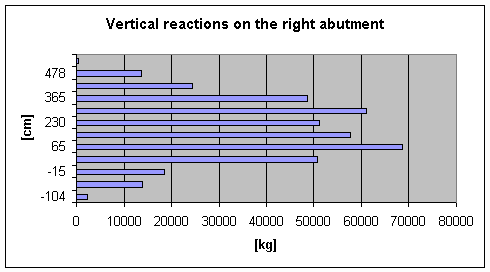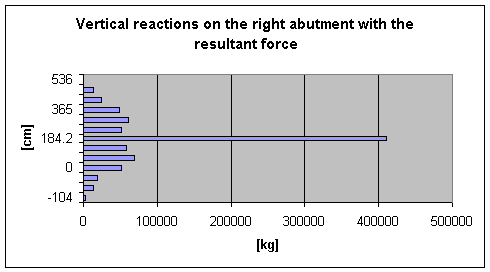In the above diagrams the level z = 0 corresponds to the level of the arch right springer. The values of the vertical reactions were evaluated at the initial section of the abutment. CREDITS: Intellectual property of this report and of the design drawings is owned by the University of Florence - Department of Civil Engineering author of the text: Prof.Eng. Andrea Vignoli – other contributes have been mentioned in related paragraphs © - General Engineering Workgroup - SOURCE: Final Design Reportback to menu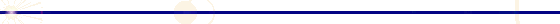GENERAL ENGINEERING - P.zzale Donatello 4 - 50132 Firenze - Italy - ph. +39 055 2345256 - fax. +39 055 2476074